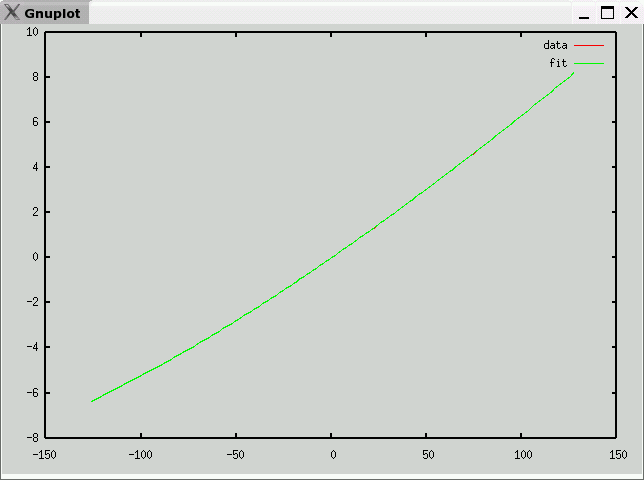# Type E Thermocouple Data and Polynomial

• Description
Ni-Cr / Cu-Ni
• Data source
National Institute of Science and Technology (NIST)
• Raw Data
• Octave format
• Curve fit
• mV -> temperature (C)
e is maximum error (in degrees C)
v1 andf v2 partition the domain into three segments, each with a polynomial fit
c is vector of coefficients: a10, a9, ... a0
Note: Each column of c corresponds to one range (<v1,v1 to v2, >v2)

`e = 0.27539v1 = -3.3060v2 = 9.8600c =   -1.8635e-03   -2.2983e-09   -1.2353e-15   -1.1959e-01    4.4509e-08   4.7181e-13   -3.4098e+00    2.5703e-07  -7.8381e-11   -5.6850e+01   -1.1748e-05   7.5325e-09   -6.1348e+02    8.4401e-05  -4.7254e-07   -4.4754e+03    4.7489e-05   2.0791e-05   -2.2343e+04   -3.0307e-03   -6.7277e-04   -7.5348e+04    1.8588e-02   1.6771e-02   -1.6423e+05   -2.4804e-01   -3.4030e-01   -2.0885e+05    1.7057e+01   1.7683e+01   -1.1769e+05    3.5498e-03  -1.5532e+00 `• temperature -> mV (range -127C-127C)
e is maximum error (in mV)
c is vector of coefficients: a10, a9, ... a0

`e = 0.00089846c =   -1.0761e-22    1.3115e-20    4.6544e-18   -5.3117e-16   -7.7836e-14    7.8256e-12    7.6493e-10   -1.2137e-07    5.0668e-05    5.8613e-02   -3.2597e-04 `Previous page: Type B
Next page: Type J